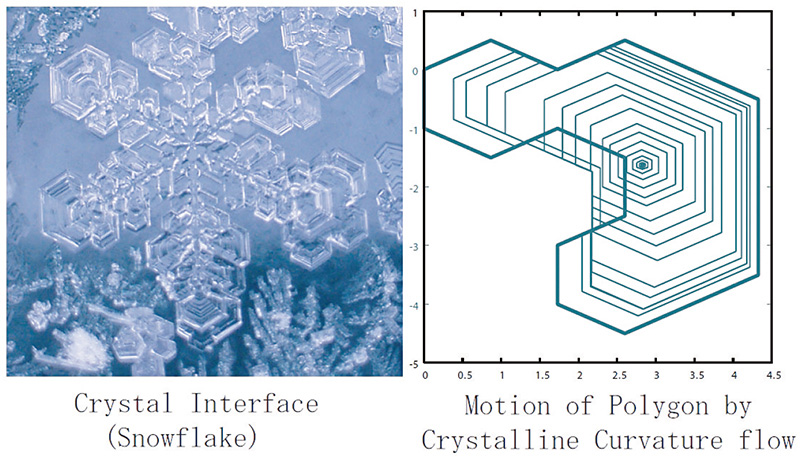# Applied Mathematics Laboratory

## Extracting the essence of phenomena and mathematically understanding it

Our laboratory expresses phenomena with equations and examines the characteristics of their solutions. In particular, we take up phenomena whose shape and condition change as time goes by, and express them with formula. To do these, we study physics or chemistry in addition to mathematics. To examine the characteristics of solutions of equations, we conduct research from various perspectives by learning necessary mathematical theories and computer simulation techniques.Faculty of Engineering Mathematical Sciences Faculty Name ISHIWATA, Tetsuya Academic Society The Mathematical Society of JapanThe Japan Society for Industrial and Applied Mathematics Keyword Nonlinear Differential equations, Numerical analyses, Computer simulations, Mathematical Models

### Study Fields

• Mathematics
• Mathematical science

### For Society

Creating mathematical model equations and analyzing them will deepen the understanding about phenomena in an objective way and help in making predictions and developing various things. Knowledge about this domain will be necessary in broad areas.

### Research Themes

• Research on mathematical modeling of nonlinear phenomena and their mathematical analyses
• Research on building numerical computation theories, simulations for practical applications and numerical simulations
• Analyses of theories on nonlinear evolution equations